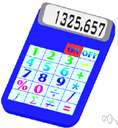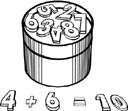Verb 1add together - determine the sum of; "Add all the people in this town to those of the neighboring town"add together, add - make an addition by combining numbers; "Add 27 and 49, please!"count, numerate, enumerate, number - determine the number or amount of; "Can you count the books on your shelf?"; "Count your change" 2add together - make an addition by combining numbers; "Add 27 and 49, please!"addarithmetic - the branch of pure mathematics dealing with the theory of numerical calculationscompute, calculate, cipher, cypher, figure, reckon, work out - make a mathematical calculation or computationfoot up, foot - add a column of numbersadd together, summate, tot, tot up, tote up, total, add up, sum, sum up, tally, add - determine the sum of; "Add all the people in this town to those of the neighboring town"